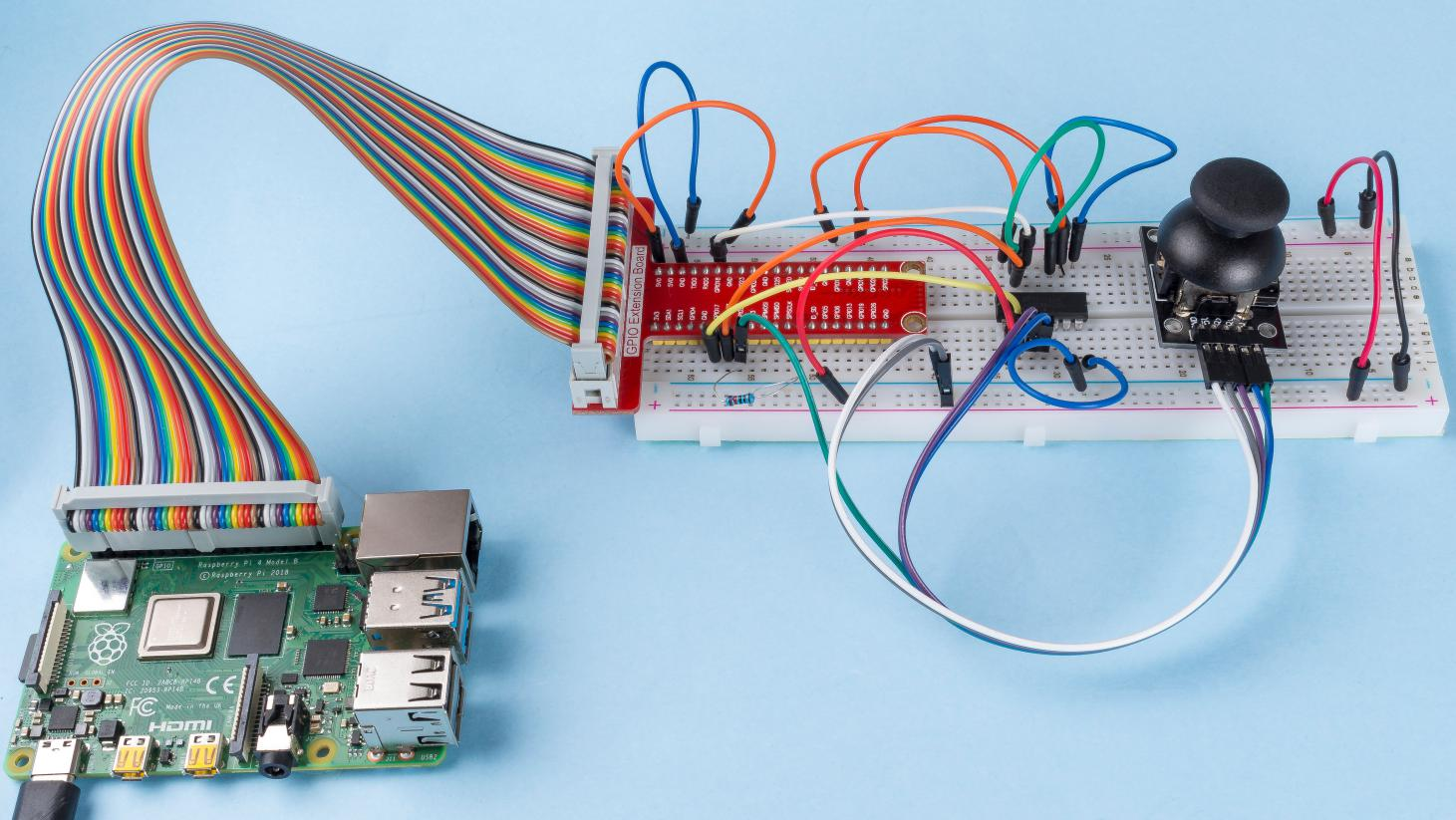# 2.1.9 Joystick¶

## Introduction¶

In this project, We’re going to learn how joystick works. We manipulate the Joystick and display the results on the screen.

## Components¶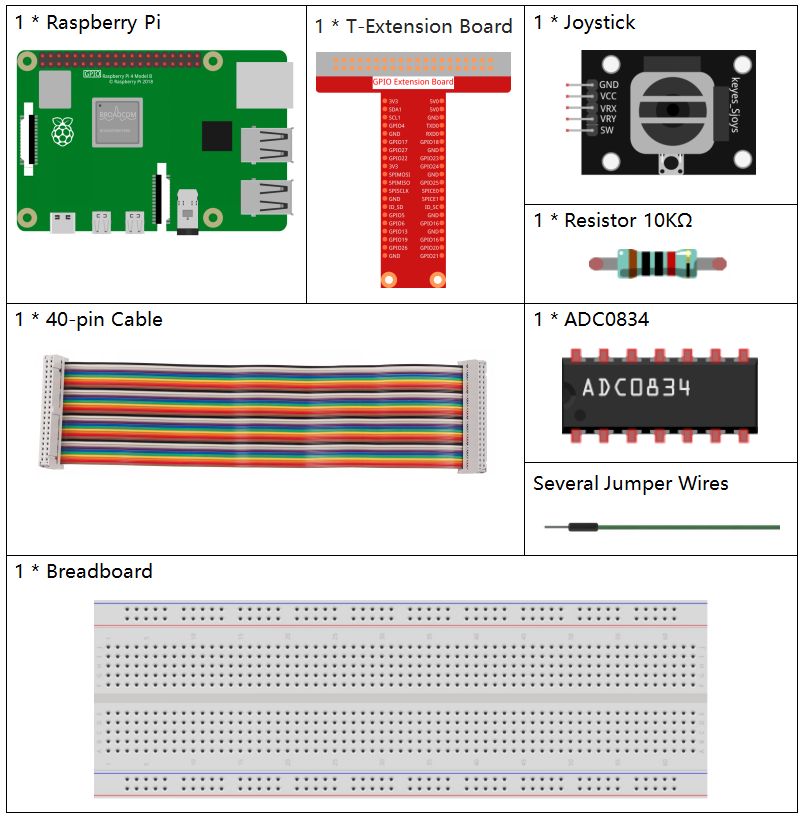## Schematic Diagram¶

When the data of joystick is read, there are some differents between axis: data of X and Y axis is analog, which need to use ADC0834 to convert the analog value to digital value. Data of Z axis is digital, so you can directly use the GPIO to read, or you can also use ADC to read.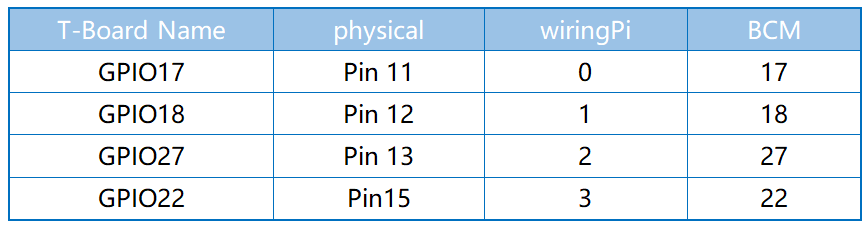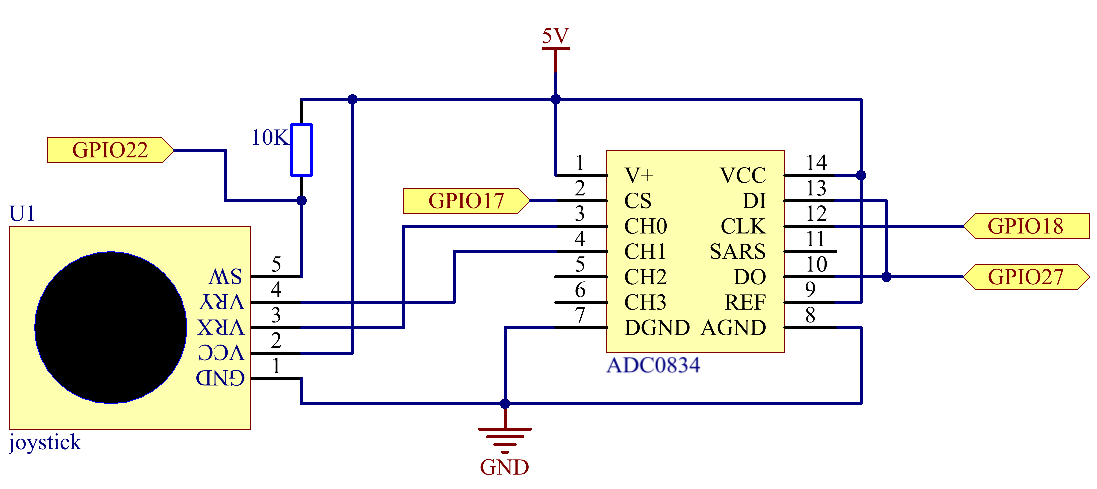## Experimental Procedures¶

Step 1: Build the circuit.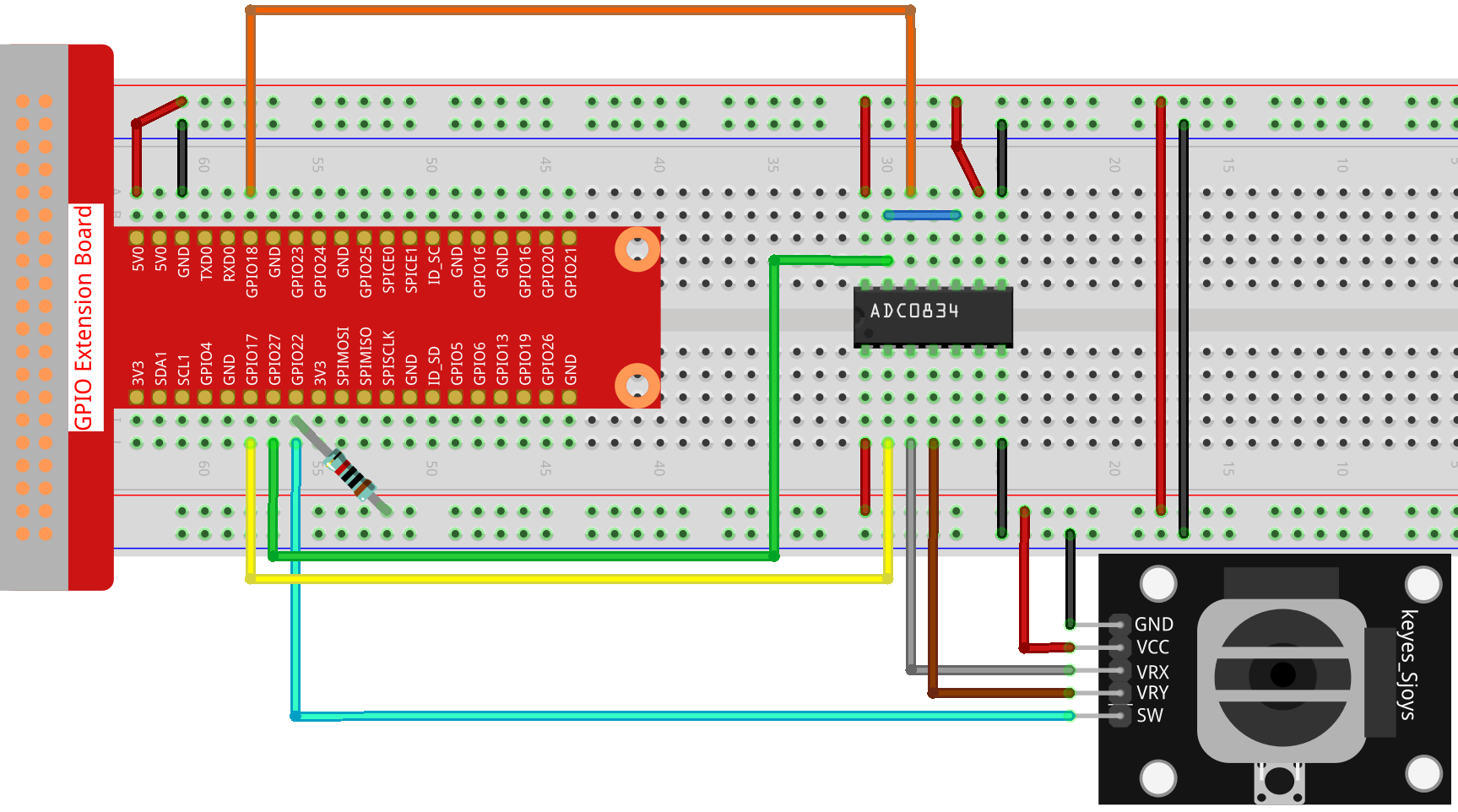Step 2: Go to the folder of the code.

```cd /home/pi/raphael-kit/python/
```

Step 3: Run.

```sudo python3 2.1.9_Joystick.py
```

After the code runs, turn the Joystick, then the corresponding values of x, y, Btn are displayed on screen.

Code

Note

You can Modify/Reset/Copy/Run/Stop the code below. But before that, you need to go to source code path like `raphael-kit/python`. After modifying the code, you can run it directly to see the effect.

```#!/usr/bin/env python3

import RPi.GPIO as GPIO
import time

BtnPin = 22

def setup():
# Set the GPIO modes to BCM Numbering
GPIO.setmode(GPIO.BCM)
GPIO.setup(BtnPin, GPIO.IN, pull_up_down=GPIO.PUD_UP)

def destroy():
# Release resource
GPIO.cleanup()

def loop():
while True:
Btn_val = GPIO.input(BtnPin)
print ('X: %d  Y: %d  Btn: %d' % (x_val, y_val, Btn_val))
time.sleep(0.2)

if __name__ == '__main__':
setup()
try:
loop()
except KeyboardInterrupt: # When 'Ctrl+C' is pressed, the program destroy() will be executed.
destroy()
```

Code Explanation

```def loop():
while True: